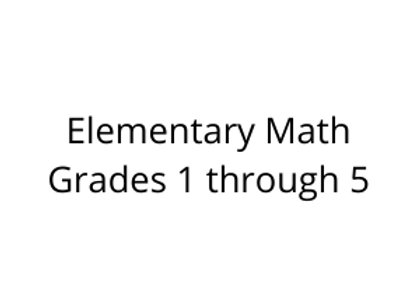# Elementary Math Grades 1 through 5

\$395.00Price
`	Grade 1 Math course focuses on addition, subtraction through the number 20, whole number relationships and place value, linear measurement, measuring lengths, and geometric shapes.	Grade 2 Math course emphasizes base-ten notation, fluency with addition and subtraction, using standard units of measure, and describing and analyzing shapes.	Grade 3 Math course focuses on multiplication and division up to the number 100, fractions, the structure of rectangular arrays and area, and two-dimensional shapes.	Grade 4 Math course emphasizes multi-digit multiplication and division, fractions, and analysis and classification of geometric figures.	Grade 5 Math course is designed to give students additional experience with basic mathematical operations (addition, subtraction, multiplication, and division). Besides working with larger whole numbers, in this course students focus on performing these mathematical operations on fractions, mixed numbers, and decimals. Students are introduced to basic algebraic and geometric concepts and many other topics to provide them with a strong mathematical foundation.`
Length